# Pandas Series: The Complete Guide

The axis labels are collectively called index. In layman’s terms, Pandas Series is nothing but the column in an excel sheet. You can control the index(label) of elements. A series label can be thought of as similar to the Python dictionary.

Series also supports vector operations. Any operation performed on the Series gets performed on every single element.

## What is Pandas Series?

Pandas Series is a one-dimensional data structure designed for a particular use case. The Series is the one-dimensional labeled array capable of holding any data type. Series is the one-dimensional labeled array capable of carrying data of any data type like integer, string, float, python objects, etc.

## How to Create a Series in Pandas?

The Pandas Series can be created from the Python list or NumPy array.

Unlike Python lists, the Series will always contain data of the same type. This makes NumPy array the better candidate for creating a pandas series.

Let’s create a series using the NumPy library.

```# app.py

import pandas as pd
import numpy as np

data = np.array(['a','b','c','d'])
seri = pd.Series(data)
print(seri)```

In the above example, we have imported two libraries which are Pandas and Numpy.

Then we used NumPy to construct the data, passed that to the series function of pandas, and created a series.

Run the above file and see the output.### Syntax

A pandas Series can be created using the following constructor.

`pandas.Series( data, index, dtype, copy)`

The data parameter takes various forms like ndarray, list, and constants.

The index parameter values must be unique and hashable, the same length as the data.

The dtype parameter is for the data type. If None, the data type will be inferred.

The copy parameter is to copy the data. The default parameter is False.

### Create an Empty Series

A primary series that can be created is an Empty Series. See the following code.

```# app.py

import pandas as pd

seri = pd.Series()
print(seri)```

See the below output.## Create a Series from ndarray

If data is a ndarray, the index passed must be the same length. If no index is passed, then by default index will be range(n) where n is array length, i.e., [0,1,2,3…. range(len(array))-1].

```# app.py

import pandas as pd
import numpy as np

data = np.array(['A','B','C','D'])
seri = pd.Series(data)
print(seri)```

In this example, we have imported the NumPy library, created a data array, and passed that data to the series function to create a Pandas Series.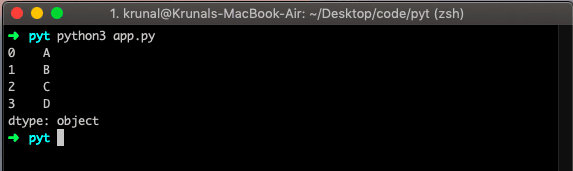If we did not pass any index, it would be assigned the indexes ranging from 0 to len(data)-1, i.e., 0 to 3.

## Provide the Indexes With Data in Series

Let’s take an example: passing the data and indexes and seeing the output.

```# app.py

import pandas as pd
import numpy as np

data = np.array(['A','B','C','D','E'])
seri = pd.Series(data, index=[18, 19, 20, 21, 22])
print(seri)```

See the output below.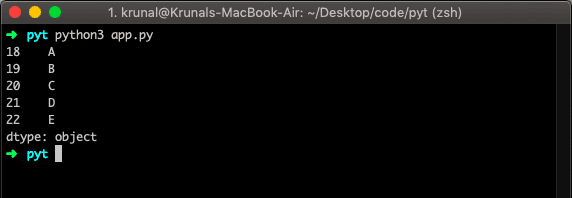### Create a Series from the dictionary

dictionary can be passed as input, and if no index is specified, then the dictionary keys are taken in the sorted order to construct an index.

If an index is passed, the values in data corresponding to the labels in the index will be pulled out.

```# app.py

import pandas as pd
import numpy as np

data = {'name' : 'krunal', 'website' : 'appdividend.com', 'role' : 'author'}
siri = pd.Series(data)
print(siri)```

We have taken the Python Dictionary as data. See the below output.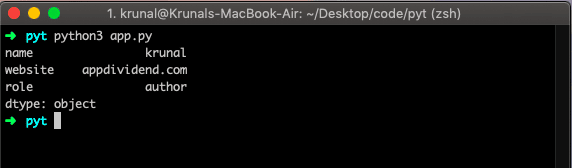### Create a Series from Scalar

If data is the scalar value, then an index must be provided.

The value will be repeated until the length of the index. See the following example.

```# app.py

import pandas as pd
import numpy as np

siri = pd.Series(21, index=[0, 1, 2, 3, 4])
print(siri)```

See the below output.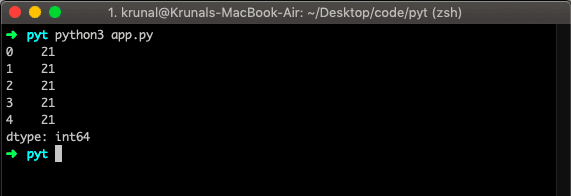## Accessing Data from Series

We can access the items through its index.

Data in the Series can be accessed similarly to that in a ndarray.

See the below example.

```# app.py

import pandas as pd
import numpy as np

data = np.array(['A','B','C','D','E'])
seri = pd.Series(data, index=[18, 19, 20, 21, 22])
print(seri)```

In the above example, we have already provided the indexes starting from 18 to 22. We get the output because the index maps to that element. Now, see the below output.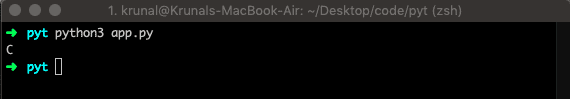That’s it.

This site uses Akismet to reduce spam. Learn how your comment data is processed.